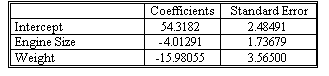### Why should I choose AnalystNotes?

Simply put: AnalystNotes offers the best value and the best product available to help you pass your exams.

##### Subject 1. Multiple Linear Regression
In financial analysis, we often need to determine the effect of more than one independent variable on a particular dependent variable. Multiple regression is a generalization of the simple linear regression analysis covered in the last session. The same ideas can be extended to analyze relationships between a dependent variable and two or more explanatory variables. If knowledge of one variable X helps us predict the value of Y, then it is natural to consider whether knowledge of several variables X1, X2, ..., Xt enables us to provide an even better prediction of the value of Y.

The population regression equation is E(Yt | X1t, X2t, ..., Xkt) = β0 + β1X1t + β2X2t + ... + βkXkt and shows the mean value of Yt associated with the given values X1t, X2t, ... Xkt of the explanatory variables.

The parameter β0 is the constant term, or Y-intercept, and measures the mean value of Yt, when all the independent variables are set to 0. The parameter β1 measures the change in the mean value of Yt corresponding to a 1-unit increase in the value of X1t, when all the other independent variables are held constant; the parameter β2 measures the change in the mean value of Yt corresponding to a 1-unit increase in the value of X2t, when all the other independent variables are held constant, and so forth.

In the population regression model, the random error term, denoted ei, is the difference between the value of the random variable Yt and the expected value E(Yt | X1t, X2t, ..., Xkt). We obtain

ei = Yt - E(Yt | X1t, X2t, ..., Xkt) = Yt - (β0 + β1X1t + β2X2t + ... + βkXkt).

After rearranging terms in the equation for the random error term ei, we obtain the equivalent expression (multiple linear regression model):

Yt = β0 + β1X1t + β2X2t + ... + βkXkt + et

where

• t = 1, 2, 3, ..., T observations.
• Yt = the dependent variable.
• Xj = the independent variables, j = 1, 2, ..., k.
• Xjt = the t-th observation on the independent variable Xj.
• β0 = the intercept of the equation. Note that in the textbook this is denoted as b0.
• β1, ..., βk = the slope coefficients for each of the independent variables. βj measures how much the dependent variable changes when the independent variable, Xjt, changes by one unit, holding all other independent variables constant. Note that in the textbook these are denoted as b0, b1, ... , bk.
• et = the error term. for any values of the independent variables, the mean value of et is 0.

We refer to both the intercept β0, and the slope coefficients, β1, ..., βk, as regression coefficients.

The population parameters β0, β1, ..., βk are unknown and are estimated using a sample of T observations on the dependent variable Y and the K independent variables X1t, X2t, ... , Xkt. Once we have estimated the parameters β0, β1, ..., βk, we obtain an estimated regression equation, which is called the sample regression equation.

y-hatt = b0 + b1x1t + b2x2t + ... + b0xkt

The value b0 is the sample estimate of the population parameter β0, the value b1 is the sample estimate of the population parameter β1, and so forth. The value y-hatt is called the fitted value of Yt or the predicted value of Yt.

Example

It is reasonable to suspect that gasoline mileage for a car is determined mainly by the car's weight and engine size. We decided to estimate the regression

Yt = b0 + b1X1t + b2X2t + et

where

• Yt = the gasoline mileage (in miles per gallon) of the t-th car.
• X1t = the engine size of the t-th car (in hundreds of cubic inches).
• X2t = the weight of the t-th car (in tons).

The following table shows the results of this linear regression using a sample of T = 10 different cars.Therefore, we obtain the estimated equation (after rounding) of y-hatt = 54.3182 - 4.0129X1t - 15.9806X2t

The predicted mileage for a car that has a 2.4-hundred-cubic-inch engine and weighs 0.9 ton is obtained by substituting the values X1t = 2.4 and X2t = -0.9 into the estimated equation. The predicted value is then 54.3182 - 4.0129 (2.4) - 15.9806 (0.9) = 30.3047, or about 30.3 miles per gallon.

The standard error column gives the standard error (the standard deviation) of the estimated regression coefficients.

We have T = 10 observations and k = 2 explanatory variables in the model, so the appropriate degrees of freedom is 10 - 2 - 1 = 7.

Learning Outcome Statements

a. formulate a multiple regression equation to describe the relation between a dependent variable and several independent variables, and determine the statistical significance of each independent variable;

b. interpret estimated regression coefficients and their p-values;

CFA® 2023 Level I Curriculum, Volume 1, Module 2